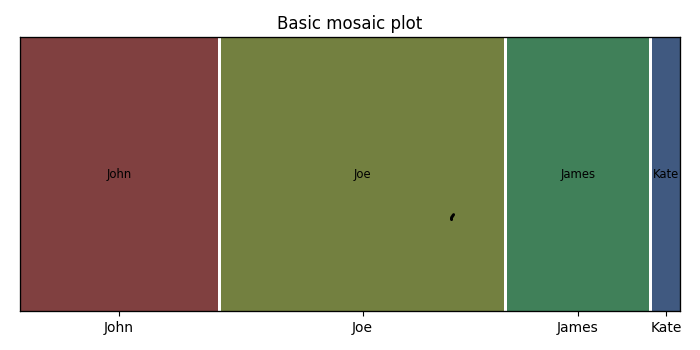# How to make a mosaic plot in Matplotlib?

To make a mosaic plot in matplotlib, we can take the following steps.

## Steps

• Set the figure size and adjust the padding between and around the subplots.

• Install statsmodel package (pip install statsmodels). It is required to create mosaic plots. statsmodels is a Python package that provides a complement to scipy for statistical computations including descriptive statistics and estimation and inference for statistical models.

• Make a dictionary for mosaic plot.

• Create a mosaic plot from a contingency table.

• To display the figure, use Show() method.

## Example

import matplotlib.pyplot as plt
from statsmodels.graphics.mosaicplot import mosaic

plt.rcParams["figure.figsize"] = [7.00, 3.50]
plt.rcParams["figure.autolayout"] = True

# Dictionary for mosaic plot
data = {'John': 7, 'Joe': 10, 'James': 5, 'Kate': 1}

# Create mosaic plot
mosaic(data, title='Basic mosaic plot')

# Display the figure
plt.show()

## Output

It will produce the following output −Updated on: 08-Oct-2021

1K+ Views